# 贝叶斯模型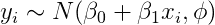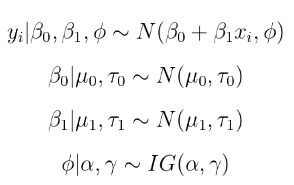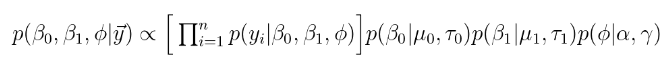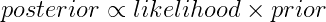R代码从指定的“真实”参数模型生成数据。我们稍后将用这个数据估计贝叶斯回归模型来检查是否可以恢复这些真实的参数。

 tphi<-rinvgamma(1, shape=a, rate=g)
tb0<-rnorm(1, m0, sqrt(t0) )
tb1<-rnorm(1, m1, sqrt(t1) )
tphi; tb0; tb1;

y<-rnorm(n, tb0 + tb1*x, sqrt(tphi))

# 吉布斯采样器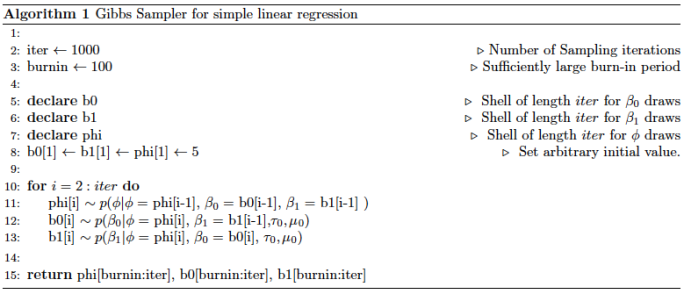# 条件后验分布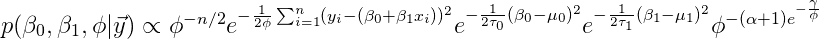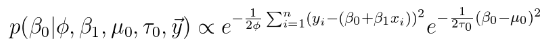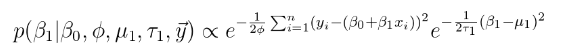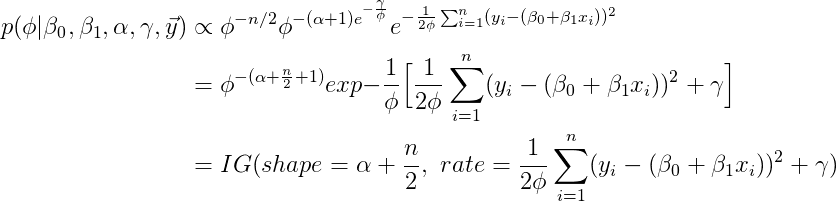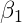​考虑网格方法。网格方法是暴力方法，从其条件后验分布进行抽样。这个条件分布只是一个函数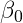​。所以我们可以评估密度​值。在R中，可以是grid = seq（-10，10，by = .001）。这个序列是点的“网格”。

 for(i in 1:length(p) ){
p[i]<- (-(1/(2*phi))*sum( (y - (grid[i]+b1*x))^2 ))  + ( -(1/(2*t0))*(grid[i] - m0)^2)
}

draw<-sample(grid, size = 1, prob = exp(1-p/max(p))) 

# 仿真结果

iter<-1000
burnin<-101
phi<-b0<-b1<-numeric(iter)
phi<-b0<-b1<-6 

### 随时关注您喜欢的主题

z <- kde2d(b0, b1, n=50)
plot(b0,b1, pch=19, cex=.4)
contour(z, drawlabels=FALSE, nlevels=10, col='red', add=TRUE)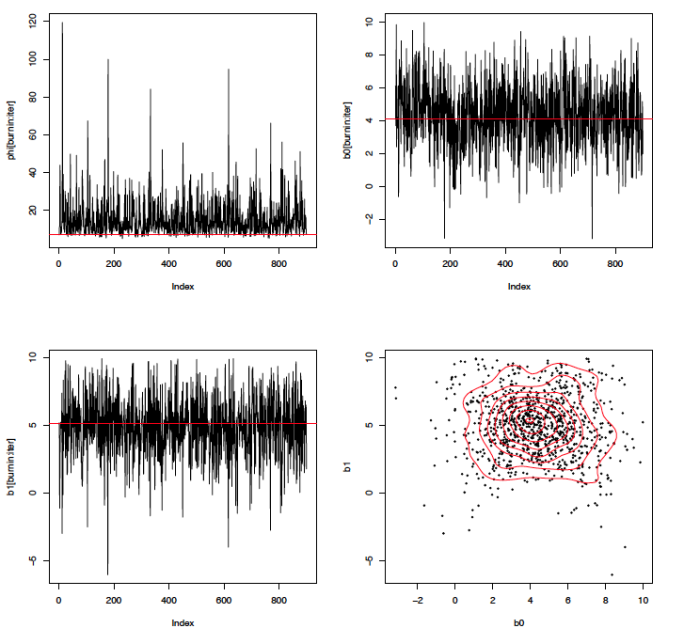Kaizong Ye拓端研究室（TRL）的研究员。

​非常感谢您阅读本文，如需帮助请联系我们！QQ在线咨询

15121130882

0571-63341498

## 关注有关新文章的微信公众号

This will close in 0 seconds